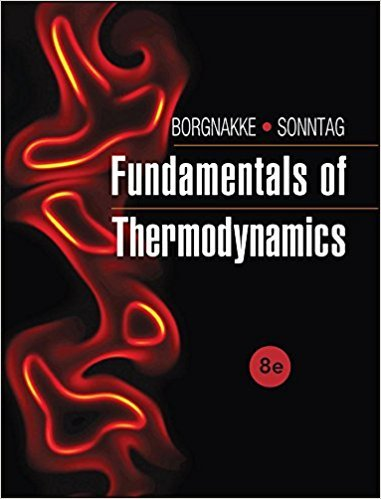×
Get Full Access to Fundamentals Of Thermodynamcs - 8 Edition - Chapter 2 - Problem 117hp
Get Full Access to Fundamentals Of Thermodynamcs - 8 Edition - Chapter 2 - Problem 117hp

×

# A container with liquid nitrogen at 100 K has aISBN: 9781118131992 58

## Solution for problem 117HP Chapter 2

Fundamentals of Thermodynamcs | 8th Edition

• Textbook Solutions
• 2901 Step-by-step solutions solved by professors and subject experts
• Get 24/7 help from StudySoup virtual teaching assistantsFundamentals of Thermodynamcs | 8th Edition

4 5 1 288 Reviews
27
0
Problem 117HP

Problem 117HP

A container with liquid nitrogen at 100 K has a cross-sectional area of 0.5 m2, as shown in Fig. P2.117. Due to heat transfer, some of the liquid evaporates, and in 1 h the liquid level drops 30 mm. The vapor leaving the container passes through a valve and a heater and exits at 500 kPa, 260 K. Calculate the volume rate of flow of nitrogen gas exiting the heater.

FIGURE P2.117Step-by-Step Solution:

Solution 117 HP

Step 1 of 3

We are required to calculate the volume rate of flow of nitrogen gas exiting the heater.

Step 2 of 3

Step 3 of 3

##### ISBN: 9781118131992

The full step-by-step solution to problem: 117HP from chapter: 2 was answered by , our top Engineering and Tech solution expert on 08/03/17, 05:05AM. The answer to “A container with liquid nitrogen at 100 K has a cross-sectional area of 0.5 m2, as shown in Fig. P2.117. Due to heat transfer, some of the liquid evaporates, and in 1 h the liquid level drops 30 mm. The vapor leaving the container passes through a valve and a heater and exits at 500 kPa, 260 K. Calculate the volume rate of flow of nitrogen gas exiting the heater.FIGURE P2.117” is broken down into a number of easy to follow steps, and 71 words. Fundamentals of Thermodynamcs was written by and is associated to the ISBN: 9781118131992. Since the solution to 117HP from 2 chapter was answered, more than 504 students have viewed the full step-by-step answer. This textbook survival guide was created for the textbook: Fundamentals of Thermodynamcs , edition: 8. This full solution covers the following key subjects: liquid, container, nitrogen, heater, area. This expansive textbook survival guide covers 7 chapters, and 1462 solutions.

Unlock Textbook Solution